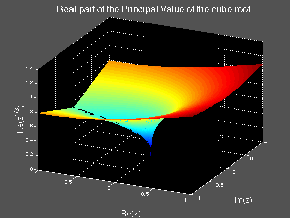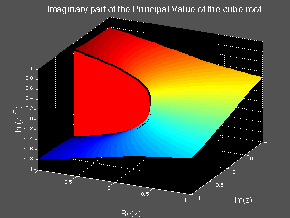18.04 | Fall 1999 | Undergraduate

# Complex Variables with Applications

Study Materials

## Branch Cuts and Branches

(All images created with MATLAB® software)

Click on pictures for more information and an image of higher resolution.

One way to get a single valued function out of a multiple valued function is to introduce branch cuts in the complex plane. These are curves joining the branch points in such a way as to prevent multiple values from arising (by eliminating paths that can go around the branch points). Then a single value can be selected (at each point in the cut plane) for the function. This then defines a branch of the multiple valued function.

We point out that the process by which a branch of a multiple valued function is constructed is somewhat arbitrary; the selection of the branch cuts is only limited by the fact that they must be there to prevent the multiple values from arising. This leaves a large amount of freedom in their selection. Thus, the branches of a multiple valued function are highly non-unique and (generally) there is nothing to make one branch special over another (excepting special reasons that may arise in specific applications and uses of complex variables).Figure 1: Real part of the Principal Value of the cube root.

Unfortunately, a price must be paid for the single valued function a branch of a multiple valued function provides. The price is that branches are discontinuous along the branch cuts. On the other hand, branches are necessary, since they provide the only practical way of actually doing computations that involve multiple valued complex functions.

We illustrate these points with the example of the principal value of the cubic root on the complex plane. This principal value is defined by the following facts: the branch cut is the negative real axis and the branch takes positive real values on the positive real axis. Equivalently, the argument of the cubic root is restricted to the range: -π/3 < θ < π/3. Figure 1 shows the real part of the principal value of the cubic root and Figure 2 shows the imaginary part. The discontinuity along the negative real axis (the branch cut) is quite evident.

Notice that, since this function can take only three values, on the complex plane cut as described here (along the negative real axis) only three possible branches can be defined. One is the principal value and the other two are just the principal value multiplied by one of the cubic roots of unity distinct from one; that is, multiplied by either: ei2π/3 or e-i2π/3

Furthermore, if we attempt to continue the function across the cut in a smooth fashion, the function (instead of taking the values given by the principal value) takes the values given by one of the other branches. This is a quite general phenomena: Any given branch of multiple valued function connects smoothly with some other branch when a path crossing a branch cut is followed. Here, as we cross the negative real axis, we will connect with one or the other of the two other branches, depending on which direction the crossing is made. In examples with many branch cuts and many possible branches, the situation can become quite confusing (the famous Minotaur labyrinth of Greek mythology might look trivial by comparison with the situations that relatively simple complex functions give rise to).Figure 2: Imaginary part of the Principal Value of the cube root.